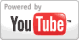Multiplying Whole Numbers - Pre-Algebra Lesson
Grade: 4 - 8 | In this math lesson, learn to multiply numbers with one or more digits, such as 56 x 7. Also learn to multiply numbers with two or more digits, such as 21 x 43, by creating two rows of products and adding the rows.Add to My DashboardMultiplication
Math Games & Activities :
GradesMath Games      (with special printable formats for classroom use)
1st - 5th Multiply by Grouping, #1Multiply by Grouping, #2Multiply by Adding
Times Tables :     of 2    of 3    of 4    of 5    of 6    of 7    of 8    of 9    of 10    of 11    of 12
Missing Factors : of 2    of 3    of 4    of 5    of 6    of 7    of 8    of 9    of 10    of 11    of 12
Times Tables, Quiz #1Times Tables, Quiz #2Times Tables, Quiz #3
Multiply Doubles       Multiply Tens       Multiply HundredsMultiply 2-digit x 1-digit
Multiplication : Word Problems, #1    Word Problems, #2Multiply 3-digit x 1-digit### Search Videos & Games